# Mechanics Basics Part 1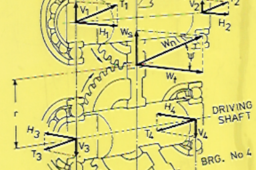This tutorial is a look at some of the basic terms and what they represent in a basic mechanics context. Most of the basic mechanics, the starting point for good mechanical design grew out of the work of Sir Isaac Newton. Newton’s work introduced the fundamental terms and principles of mechanical design by application of the physical laws of the universe.

From regularly looking at some of the questions posted here on GrabCad, even taking into consideration that for many here, English is not their main language exactly what the person asking a particular question actually wants to know, is sometimes quite hard to deduce. Some things should be universal in most languages especially when working with the ISO standard S. I. Units.

1. ### Step 1:

The first definition is the concept of MASS. MASS is a fixed quantity. It is the measure of the quantity of matter contained in a body. In the S.I system of units the standard used in engineering is the kilogram which has the abbreviation of Kg. A Kg of any substance is a constant property, mass stays the same regardless where in the universe it may be. There is another property of a body, WEIGHT that can also be measured in Kg that is not the same as MASS in spite of often described in the same terminology, kilograms. Weight is the measure of force on a body under the influence of the force of gravity, on earth a one Kg mass will have a weight of one Kg. The same one Kg mass weighed on the moon, with it’s lower gravity will weigh less than one Kg.

The first fundamental mechanics concept to get sorted in your mind :

1. Mass is a fixed quantity of matter contained in a body
2. Weight is the measure of the force gravity exerts on a body

Mass and weight, though often given the same unit of measure, Kg, are not the same thing :

Mass is fixed. Weight is a variable

Statics

Statics is the analysis of the forces acting upon structures and bodies at rest. So the starting point is first understanding exactly what a FORCE is. Back in the 1660’s this clever dude Sir Isaac Newton came up with some fundamental rules of the physical universe that became the starting point for much of the science and technology that we have today. Along with coming up with optics, a big player in the development of mathematics, in particular calculus, the basic rules of gravity he gave us this simple but extremely important and useful formula that engineering has made good use of, from bridges and other structures, all of our mechanical machines, even sending rockets and satellites into space. This useful formula is the well known relationship between mass, acceleration, and force :

FORCE = MASS x ACCELERATION

It is totally impossible to be any sort of an engineer without knowing this formula, understanding this formula and knowing how to make use of this formula. If you can’t understand this engineering is not for you.

2. ### Step 2: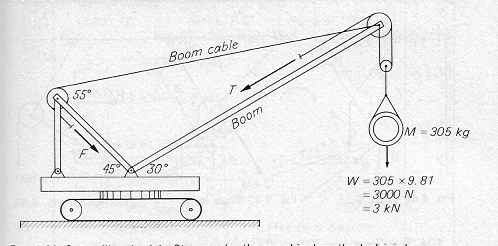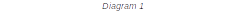Diagram 1 shows a typical problem we would use a statics analysis to solve. We will not actually solve this at this time, but we will use this as an example of applying the formula

FORCE = Mass x Acceleration

From diagram 1 we see that this simple crane is required to lift a mass of 305 Kg. From the definition of mass and weight we learned previously mass is a fixed quantity of matter and is a constant. So we can’t use mass as the force that will load up the structure. It is this loading of the structure we are after, as to properly engineer a machine like this, that will work and not break when in use, calculating the loading places on this machine by lifting 305 Kg is our starting point. We need to work out the force, this fixed quantity of mass places, on the machine. We need to work out the force that this mass working under the influence of gravity produces.

We know that : F = M x A , we have been given the mass involved, so all we need to know is the acceleration applied to this mass, by gravity. We could, with the aid of sensitive instruments, measure the actual gravitational effect at our location. This gravity has slightly different values at different places on the planet and for practical engineering purposes would be a nuisance, so instead, for our purpose for the value of the acceleration, we will use the universal gravitational constant.

The value of this constant is - 9.81 m/s2 so this is the value we will use in our calculations.

F = M x A → = 305 x 9.81 = 2992.05 N which for our calculation purpose 3000 N is near enough

The standard unit used with the S.I. units system for force is the Newton, named after Sir Isaac. This is abbreviated to N. The Newton is defined within S.I units as the force produced by a 1 Kg mass accelerated by 1 m/sec2. The S.I system is based on the underlying unit for mass, the kilogram, for distance, the metre, for time, the second. The entire units in the S.I. system are all derived from Kg, m, sec. Often we will be working in mm or tonnes or minuets, converting everything back into the Kg, m, second with the units in our answer being derived from Kg, m, sec before we start is often a good way to start as the units used in our answer came from this standard S.I approach to units.

3. ### Step 3:

One very useful tool we make use of, to analyze mechanics problems, is vector diagrams. These diagrams are a graphical representation of the mechanics problem we desire to solve. The vectors we draw in these diagrams represent the action and value of the forces involved. The length of these vectors is a scalar value that represents the magnitude of the force, our vector represents, the direction of the vector, represents the line of action of the force and we connect the vectors to enable the diagram to find the solution we are seeking. In the pre-cad era when I was taught how to apply vector diagrams to solve engineering problems, depending on the required accuracy of the obtained result, we could draw these vector diagrams, to scale, and as accurately as we could, obtaining our answer by carefully measuring the resulting diagram. For a more accurate answer we would apply a bit of trigonometry to calculate the result. In the CAD era we now find ourselves in, the CAD tool ,can give us the same accuracy as the trigonometry calculation method. We will now look at a simple example, and use vector diagrams to analyze the forces involved and to obtain some results we would need to design a real world working solution that we know will be up to the task in hand.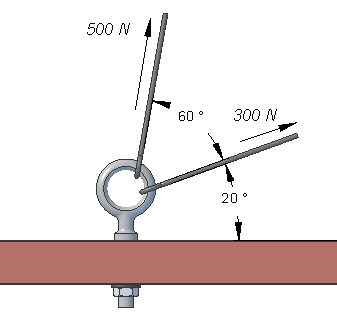This image, Diagram 3 shows the solution obtained using the parallelogram of forces method. The two forces are drawn acting through a common point at the angles obtained from the space diagram. I drew these to scale, 10mm = 100 N. The resultant force is obtained by drawing in the diagonal from the parallelogram constructed from lines of equal magnitude and parallel to the vectors representing the two forces. I then measured this resultant vector and found it to be 70 mm long. By applying the same scale to the resultant we find it to represent a force with a magnitude of 700 N. I measure this angle in relationship to the line representing the floor and find it to be acting at 58.21 deg from the floor. As I produced this diagram as a CAD sketch the result I obtained would have the same accuracy as calculating the answer using trigonometry.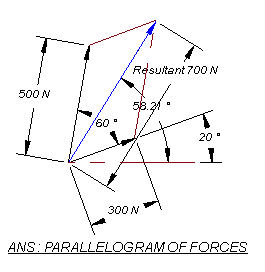The image, Diagram 4, shows using the similar triangle of forces approach. I have placed a line to represent the floor. Starting at one end of this line a draw the first vector 30 mm long at 20 deg to the floor to represent the 300 N force. At the end of this vector I draw another vector 50 mm long at 60 deg from the first vector to represent the 500 N force. The resultant is represented by the blue line that closes the triangle. When measured it is 70 mm long and acting at 58.21 deg to the floor, the same answer obtained from the parallelogram method. Looking at the basic geometry for the processes we would expect to to get the same result.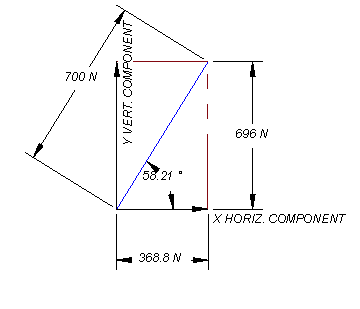Another thing besides finding the resultant force, is to resolve this resultant into X and Y components ( horizontal and vertical forces ) as in Diagram 5. We could use these component forces to further analyze the eye-bolt. We could use this to check the tensile, shear and bending stress placed onto this part.

This is a simple example. Vector diagrams are a useful tool for analyzing many engineering problems. Even if you may choose to analyze a particular object a good understanding vector diagrams and how loading's on a particular item interact can be of assistance in performing FEM analysis that will produce a correct answer. Only by applying all of the loading's correctly will we have the possibility of getting a correct result.